# ML Aggarwal Class 10 Solutions for ICSE Maths Chapter 17 Mensuration Ex 17.5

## ML Aggarwal Class 10 Solutions for ICSE Maths Chapter 17 Mensuration Ex 17.5

ML Aggarwal Class 10 Solutions for ICSE Maths Chapter 17 Mensuration Ex 17.5

Question 1.
The diameter of a metallic sphere is 6 cm. The sphere is melted and drawn into a wire of uniform cross-section. If the length of the wire is 36 m, find its radius.
Solution:Question 2.
The radius of a sphere is 9 cm. It is melted and drawn into a wire of diameter 2 mm. Find the length of the wire in metres.
Solution:Question 3.
A solid metallic hemisphere of radius 8 cm is melted and recast into a right circular cone of base radius 6 cm. Determine the height of the cone.
Solution: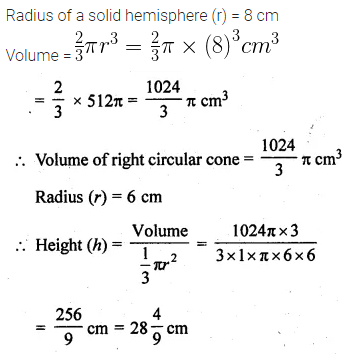Question 4.
A rectangular water tank of base 11 m x 6 m contains water up to a height of 5 m. if the water in the tank is transferred to a cylindrical tank of radius 3.5 m, find the height of the water level in the tank.
Solution:Question 5.
The rainwater from a roof of dimensions 22 m x 20 m drains into a cylindrical vessel having a diameter of base 2 m and height; 3.5 m. If the rainwater collected from the roof just fill the cylindrical vessel, then find the rainfall in cm.
Solution: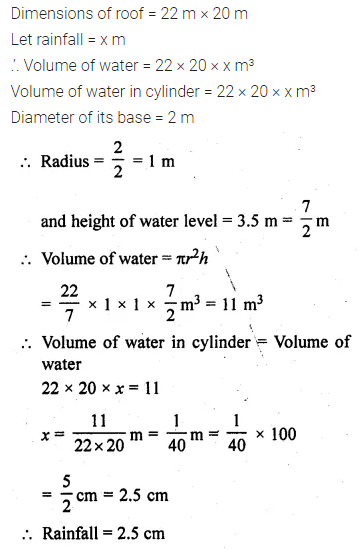Question 6.
The volume of a cone is the same as that of the cylinder whose height is 9 cm and diameter 40 cm. Find the radius of the base of the cone if its height is 108 cm.
Solution: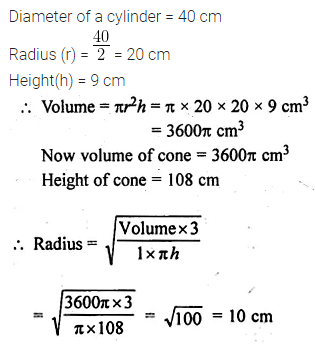Question 7.
Eight metallic spheres, each of radius 2 cm, are melted and cast into a single sphere. Calculate the radius of the new (single) sphere.
Solution:Question 8.
A metallic disc, in the shape of a right circular cylinder, is of height 2.5 mm and base radius 12 cm. Metallic disc is melted and made into a sphere. Calculate the radius of the sphere.
Solution:Question 9.
Two spheres of the same metal weigh 1 kg and 7 kg. The radius of the smaller sphere is 3 cm. The two spheres are melted to form a single big sphere. Find the diameter of the big sphere.
Solution:Question 10.
A hollow copper pipe of inner diameter 6 cm and outer diameter 10 cm is melted and changed into a solid circular cylinder of the same height as that of the pipe. Find the diameter of the solid cylinder.
Solution:Question 11.
A solid sphere of radius 6 cm is melted into a hollow cylinder of uniform thickness. If the external radius of the base of the cylinder is 4 cm and height is 72 cm, find the uniform thickness of the cylinder.
Solution:Question 12.
A hollow metallic cylindrical tube has an internal radius of 3 cm and height 21 cm. The thickness of the metal of the tube is $$\\ \frac { 1 }{ 2 }$$ cm. The tube is melted and cast into a right circular cone of height 7 cm. Find the radius of the cone correct to one decimal place.
Solution: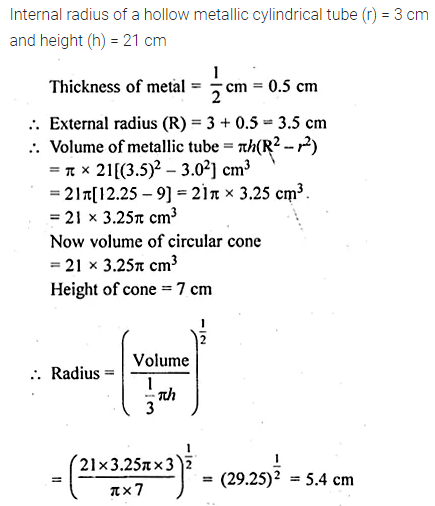Question 13.
A hollow sphere of internal and external diameters 4 cm and 8 cm respectively, is melted into a cone of base diameter 8 cm. Find the height of the cone. (2002)
Solution:Question 14.
A well with inner diameter 6 m is dug 22 m deep. Soil taken out of it has been spread evenly all round it to a width of 5 m to form an embankment. Find the height of the embankment.
Solution: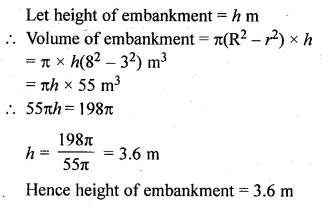Question 15.
A cylindrical can of internal diameter 21 cm contains water. A solid sphere whose diameter is 10.5 cm is lowered into the cylindrical can. The sphere is completely immersed in water. Calculate the rise in water level, assuming that no water overflows.
Solution:Question 16.
There is water to a height of 14 cm in a cylindrical glass jar of radius 8 cm. Inside the water, there is a sphere of diameter 12 cm completely immersed. By what height will the water go down when the sphere is removed?
Solution: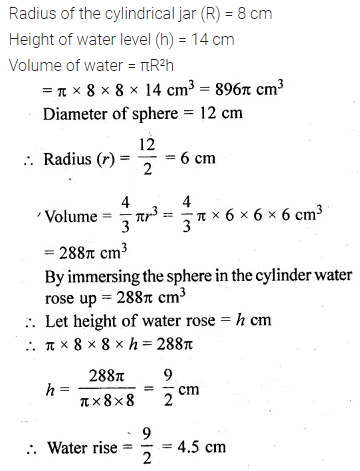Question 17.
A vessel in the form of an inverted cone is filled with water to the brim. Its height is 20 cm and diameter is 16.8 cm. Two equal solid cones are dropped in it so that they are fully submerged. As a result, one-third of the water in the original cone overflows. What is the volume of each of the solid cone submerged? (2002)
Solution: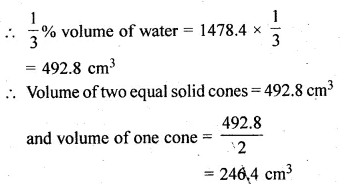Question 18.
A solid metallic circular cylinder of radius 14 cm and height 12 cm is melted and recast into small cubes of edge 2 cm. How many such cubes can be made from the solid cylinder?
Solution:Question 19.
How many shots each having diameter 3 cm can be made from a cuboidal lead solid of dimensions 9 cm × 11 cm × 12 cm?
Solution:Question 20.
How many spherical lead shots of diameter 4 cm can be made out of a solid cube of lead whose edge measures 44 cm?
Solution:Question 21.
Find the number of metallic circular discs with 1.5 cm base diameter and height 0.2 cm to be melted to form a circular cylinder of height 10 cm and diameter 4.5 cm.
Solution:Question 22.
A solid metal cylinder of radius 14 cm and height 21 cm is melted down and recast into spheres of radius 3.5 cm. Calculate the number of spheres that can be made.
Solution:Question 23.
A metallic sphere of radius 10.5 cm is melted and then recast into small cones, each of radius 3.5 cm and height 3 cm. Find the number of cones thus obtained. (2005)
Solution:Question 24.
A certain number of metallic cones each of radius 2 cm and height 3 cm are melted and recast in a solid sphere of radius 6 cm. Find the number of cones. (2016)
Solution:Question 25.
A vessel is in the form of an inverted cone. Its height is 11 cm and the radius of its top, which is open, is 2.5 cm. It is filled with water up to the rim. When some lead shots, each of which is a sphere of radius 0.25 cm, are dropped into the vessel, $$\\ \frac { 2 }{ 5 }$$ of the water flows out. Find the number of lead shots dropped into the vessel. (2003)
Solution:Question 26.
The surface area of a solid metallic sphere is 616 cm². It is melted and recast into smaller spheres of diameter 3.5 cm. How many such spheres can be obtained? (2007)
Solution:Question 27.
The surface area of a solid metallic sphere is 1256 cm². It is melted and recast into solid right circular cones of radius 2.5 cm and height 8 cm. Calculate
(i) the radius of the solid sphere.
(ii) the number of cones recasts. (Use π = 3.14).
Solution:Question 28.
Water is flowing at the rate of 15 km/h through a pipe of diameter 14 cm into a cuboid pond which is 50 m long and 44 m wide. In what time will the level of water in the pond rise by 21 cm?
Solution: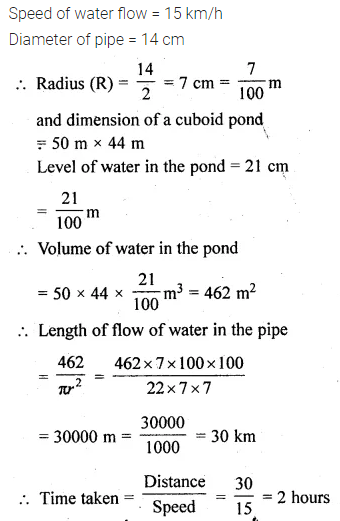Question 29.
A cylindrical can whose base is horizontal and of radius 3.5 cm contains sufficient water so that when a sphere is placed in the can, the water just covers the sphere. Given that the sphere just fits into the can, calculate:
(i) the total surface area of the can in contact with water when the sphere is in it.
(ii) the depth of the water in the can before the sphere was put into the can. Given your answer as proper fractions.
Solution: